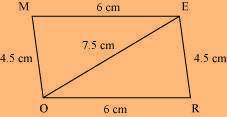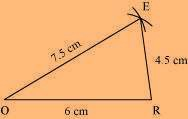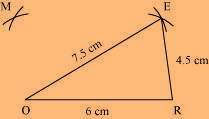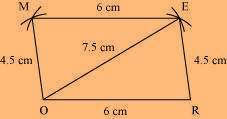# Construct the following quadrilateral: Parallelogram MORE. OR = 6 cm

Solution

RE = 4.5 cm EO = 7.5

We know that opposite sides of a parallelogram are equal in length and also these are parallel to each other. i.e., ME = OR, MO = ERThe rough sketch of the parallelogram MORE can be drawn as follows.

(1) ∆ EOR can be constructed by using the given measurements as per the steps(2) Vertex M is 4.5 cm away from vertex O and 6 cm away from vertex E.

Hence, while taking O and E as centres, draw arcs of 4.5 cm radius and 6 cm radius respectively.

These will intersect each other at point M.(3) Join M to O and E.MORE is the needed parallelogram.